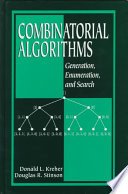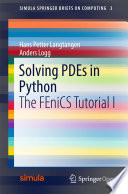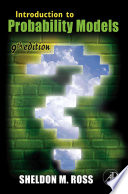### Selected Topics in Mathematics

#### Course Description

The subject qualifies stuents in deeper understanding of fundamental modern mathematical structures in the area of discrete mathematics, combinatorics, algorithm analysis, probability, numerical mathematics and mathematical modelling.

#### Forms of Teaching

Lectures

4 hours of lectures a week. The lecturer is going to follow the lecture materials.

Independent assignments

Each student will get a project in one of the three major areas: discrete mathematics, probability or mathematical modelling. The project includes analysis of a problem, studying as well as solving it using the computer.

Continuous Assessment Exam
Type Threshold Percent of Grade Threshold Percent of Grade
Seminar/Project 0 % 25 % 0 % 25 %
Mid Term Exam: Written 0 % 25 % 0 %
2. Mid Term Exam: Written 0 % 25 % 0 %
Final Exam: Written 0 % 25 %
Exam: Written 0 % 75 %

#### Week by Week Schedule

1. Introduction to the main topics and challenges. Student project presentations. Generation of all subsets of a given set. Different lexicographical orderings.
2. Generation of all k-subsets, all functions, injective and surjective functions. Adding constraints and software solutions.
3. Permutations. The successor function. Permutations without fixed elements. Integer partitions.
4. Bell's numbers. Stirling numbers. Software solutions. Combinatorial designs.
5. Introduction to mathematical modelling. Weak formulation of a mathematical model. Introduction to the finite element method.
6. Implementation of the finite element method for the 1D problem. Methods of solving linear systems.
7. Finite element method for a two-dimensional Poisson problem. GMSH and non-trivial domains.
8. Midterm exam
9. Weak formulation and finite element solvers for several models from practice.
10. Parameter tables for designs. Construction strategies. Difference sets. Introduction to Monte Carlo simulations.
11. Introduction to Markov chains. Transition probability matrix. Chapman-Kolmogorov Equations.
12. Simulation of Markov chains. Classification of States.
13. Limiting distribution. Stationary distribution. Ergodic theorem.
14. Project work and presentation.
15. Final exam

#### Study Programmes

Courses for exceptionally successful students (4. semester) (6. semester)
Courses for exceptionally successful students (4. semester) (6. semester)

#### LiteratureDonald L. Kreher, Douglas R. Stinson (1998.), Combinatorial Algorithms, CRC PressHans Petter Langtangen, Anders Logg (2017.), Solving PDEs in Python, SpringerSheldon M. Ross (2007.), Introduction to Probability Models, Academic Press

#### General

ID 214698
Summer semester
6 ECTS
L0 English Level
L1 e-Learning
60 Lectures
0 Seminar
0 Exercises
0 Laboratory exercises
0 Project laboratory